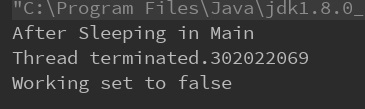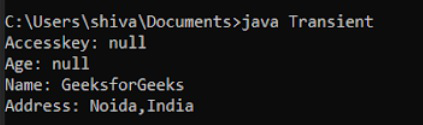# Difference between volatile and transient keywords in Java

• Last Updated : 16 Aug, 2020

Just like any other programming language, Java has a set of keywords which are reserved and have a special meaning. In this article, we will see the difference between the keywords volatile and transient.

Before getting into the differences, let us first understand what each of them actually means.

Attention reader! Don’t stop learning now. Get hold of all the important Java Foundation and Collections concepts with the Fundamentals of Java and Java Collections Course at a student-friendly price and become industry ready. To complete your preparation from learning a language to DS Algo and many more,  please refer Complete Interview Preparation Course.

Volatile:

The Volatile keyword is used to mark the JVM and thread to read its value from primary memory and not utilize cached value present in the thread stack. It is used in concurrent programming in java.

## Java

 `class` `GFG ``extends` `Thread {`` ` `    ``// using volatile``    ``volatile` `boolean` `working = ``true``;`` ` `    ``// if non-volatile it will``    ``// sleep in main and``    ``// runtime error will coocur``    ``public` `void` `run()``    ``{``        ``long` `count = ``0``;``        ``while` `(working) {``            ``count++;``        ``}`` ` `        ``System.out.println(``"Thread terminated."``                           ``+ count);``    ``}`` ` `    ``// Driver code``    ``public` `static` `void` `main(String[] args)``        ``throws` `InterruptedException``    ``{``        ``GFG test = ``new` `GFG();``        ``test.start();``        ``Thread.sleep(``100``);``        ``System.out.println(``"After Sleeping in Main"``);``        ``test.working = ``false``;``        ``test.join();``        ``System.out.println(``"Working set to "``                           ``+ test.working);``    ``}``}`

Output:Volatile Code Output

Transient:

The Transient keyword is used with the instance variable to eliminate it from the serialization process. During serialization, the value of the transient field or variable is not saved.

## Java

 `import` `java.io.*;`` ` `class` `Test ``implements` `Serializable {`` ` `    ``// Making Accesskey transient for security``    ``transient` `String accessKey;`` ` `    ``// Making age transient as age can be``    ``// calculated from Date of Birth``    ``// and current date.``    ``transient` `int` `age;`` ` `    ``// serialize other fields``    ``String name, address;``    ``public` `Test(String accessKey, ``int` `age,``                ``String name, String address)``    ``{``        ``this``.accessKey = accessKey;``        ``this``.age = age;``        ``this``.name = name;``        ``this``.address = address;``    ``}``}`` ` `public` `class` `GFG {``    ``public` `static` `void` `main(String[] args)``        ``throws` `Exception``    ``{``        ``ObjectInputStream in``            ``= ``new` `ObjectInputStream(``                ``(``new` `FileInputStream(``                    ``"login_details.txt"``)));``        ``Test obj = (Test)in.readObject();`` ` `        ``/* Transient variable will be shown ``        ``null due to security reasons.*/``        ``System.out.println(``"Accesskey: "``                           ``+ obj.accessKey);``        ``System.out.println(``"Age: "``                           ``+ obj.age);``        ``System.out.println(``"Name: "``                           ``+ obj.name);``        ``System.out.println(``"Address: "``                           ``+ obj.address);``    ``}``}`

Output:Transient Code Output

The following table describes the differences:

My Personal Notes arrow_drop_up